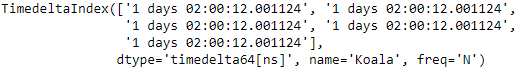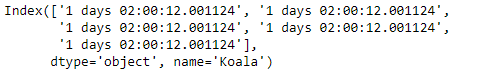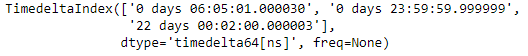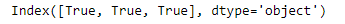Related Articles
Python | Pandas TimedeltaIndex.astype()
• Last Updated : 27 Jan, 2019

Python is a great language for doing data analysis, primarily because of the fantastic ecosystem of data-centric python packages. Pandas is one of those packages and makes importing and analyzing data much easier.

Pandas` TimedeltaIndex.astype()` function create an Index with values cast to dtypes. The class of a new Index is determined by dtype. When conversion is impossible, a ValueError exception is raised.

Syntax : TimedeltaIndex.astype(dtype, copy=True)

Parameters :
dtype : numpy dtype or pandas type
copy : bool, default True
By default, astype always returns a newly allocated object. If copy is set to False and internal requirements on dtype are satisfied, the original data is used to create a new Index or the original Index is returned.

Return : Index object

Example #1: Use `TimedeltaIndex.astype()` function to cast the values of the TimedeltaIndex object to ‘str’.

 `# importing pandas as pd``import` `pandas as pd`` ` `# Create the first TimedeltaIndex object``tidx ``=` `pd.TimedeltaIndex(start ``=` `'1 days 02:00:12.001124'``,``                       ``periods ``=` `5``, freq ``=` `'N'``, name ``=` `'Koala'``)`` ` `# Print the TimedeltaIndex object``print``(tidx)`

Output :Now we will use the `TimedeltaIndex.astype()` function to cast the value to string.

 `# cast the data values to string format.``tidx.astype(``'str'``)`

Output :As we can see in the output, the `TimedeltaIndex.astype()` function has cast the values of the tidx object to the desired format.

Example #2: Use `TimedeltaIndex.astype()` function to cast the values of the TimedeltaIndex object to ‘bool’.

 `# importing pandas as pd``import` `pandas as pd`` ` `# Create the TimedeltaIndex object``tidx ``=` `pd.TimedeltaIndex(data ``=` `[``'06:05:01.000030'``, ``'+23:59:59.999999'``,``                                              ``'22 day 2 min 3us 10ns'``])`` ` `# Print the TimedeltaIndex object``print``(tidx)`

Output :Now we will use the `TimedeltaIndex.astype()` function to cast the value to bool type.

 `# cast the data values to bool type.``tidx.astype(``'bool'``)`

Output :As we can see in the output, the `TimedeltaIndex.astype()` function has cast the values of the tidx object to the desired format.

Attention geek! Strengthen your foundations with the Python Programming Foundation Course and learn the basics.

To begin with, your interview preparations Enhance your Data Structures concepts with the Python DS Course.

My Personal Notes arrow_drop_up